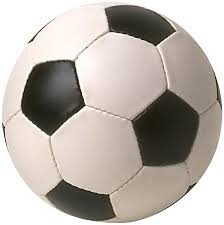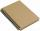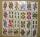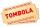# Tournament

How many matches will be played in a football tournament in which there are two groups of 5 teams if one match is played in groups with each other and the group winners play a match for the overall winner of the tournament?

Correct result:

n =  21

#### Solution:

$C_{{ 2}}(5)=\dbinom{ 5}{ 2}=\dfrac{ 5! }{ 2!(5-2)!}=\dfrac{ 5 \cdot 4 } { 2 \cdot 1 }=10 \ \\ \ \\ n_{1}={ { 5 } \choose 2 }=10 \ \\ \ \\ n=2 \cdot \ n_{1}+1=2 \cdot \ 10+1=21$Our examples were largely sent or created by pupils and students themselves. Therefore, we would be pleased if you could send us any errors you found, spelling mistakes, or rephasing the example. Thank you!

Leave us a comment of this math problem and its solution (i.e. if it is still somewhat unclear...):Be the first to comment!Tips to related online calculators
Would you like to compute count of combinations?

## Next similar math problems:

• TournamentSix teams entered the basketball tournament. How many matches will be played if each team has to play one match with each other?There are 15 boys and 12 girls at the graduation party. Determine how many four couples can be selected.
• Two groupsThe group of 10 girls should be divided into two groups with at least 4 girls in each group. How many ways can this be done?
• SalamiHow many ways can we choose 5 pcs of salami if we have 6 types of salami for 10 pieces and one type for 4 pieces?How many ways can we thread 4 red, 5 blue, and 6 yellow beads onto a thread?
• Three workplacesHow many ways can we divide nine workers into three workplaces if they need four workers in the first workplace, 3 in the second workplace and 2 in the third?
• Word MATEMATIKAHow many words can be created from the word MATEMATIKA by changing the order of the letters, regardless of whether or not the words are meaningful?
• Combinations of sweatersI have 4 sweaters two are white, 1 red and 1 green. How many ways can this done?
• ClassroomOf the 26 pupils in the classroom, 12 boys and 14 girls, four representatives are picked to the odds of being: a) all the girls b) three girls and one boy c) there will be at least two boys
• Boys and girlsThere are 11 boys and 18 girls in the classroom. Three pupils will answer. What is the probability that two boys will be among them?
• Sum or productWhat is the probability that two dice fall will have the sum 7 or product 12?
• Three redsWhat is the probability that when choosing 3 carats from seven carats, all 3 reds will be red?
• CardsFrom a set of 32 cards we randomly pull out three cards. What is the probability that it will be seven king and ace?
• RaffleThere are 200 draws in the raffle, but only 20 of them win. What is the probability of at least 4 winnings for a group of people who have bought 5 tickets together?
• Boys and girlsThere are eight boys and nine girls in the class. There were six children on the trip from this class. What is the probability that left a) only boys b) just two boys
• Families 2There are 729 families having 6 children each. The probability of a girl is 1/3 and the probability of a boy is 2/3. Find the the number of families having 2 girls and 4 boys.
• STRESSED wordEach letter in STRESSED is printed on identical cards, one letter per card and assembled in random order. Calculate the probability that the cards spell DESSERTS when assembled.